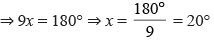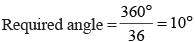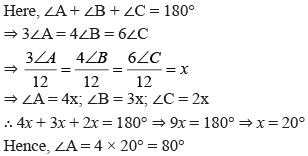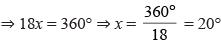Courses

# Olympiad Test : Geometry - 2

## 20 Questions MCQ Test Maths Olympiad Class 6 | Olympiad Test : Geometry - 2

Description
This mock test of Olympiad Test : Geometry - 2 for Class 6 helps you for every Class 6 entrance exam. This contains 20 Multiple Choice Questions for Class 6 Olympiad Test : Geometry - 2 (mcq) to study with solutions a complete question bank. The solved questions answers in this Olympiad Test : Geometry - 2 quiz give you a good mix of easy questions and tough questions. Class 6 students definitely take this Olympiad Test : Geometry - 2 exercise for a better result in the exam. You can find other Olympiad Test : Geometry - 2 extra questions, long questions & short questions for Class 6 on EduRev as well by searching above.
QUESTION: 1

Solution:
QUESTION: 2

Solution:
QUESTION: 3

### How many parts does a triangle have?

Solution:
QUESTION: 4

The angle of a triangle are in the ratio 2 : 3 : 4. The smallest angle is

Solution:

Here,
2x + 3x + 4x = 180°Smallest angle = 2x = 2 × 20° = 40°

QUESTION: 5

One of the base angle of an isosceles triangle is 65°. The vertical angle is

Solution:

Vertical angle = 180° – (65° + 65°)
= 180° – 130° = 50°

QUESTION: 6

Each angle of an equilateral triangle is

Solution:
QUESTION: 7

An angle measuring 360° is

Solution:
QUESTION: 8

The measure of a straight angle is

Solution:
QUESTION: 9

An angle measuring 205° is

Solution:
QUESTION: 10

If there are 36 spokes in a bicycle wheel then the angle between a pair of adjacent spokes is

Solution:QUESTION: 11

The minimum number of points of intersection of three lines in a plane is

Solution:
QUESTION: 12

Two planes intersect

Solution:
QUESTION: 13

One of the acute angle of a right triangle is 55°. What is the other acute angle?

Solution:

Other angle = 180° – (90° + 55°)
= 180° – 145° = 35°

QUESTION: 14

In a ∆ABC, 3∠A = 4∠B = 6∠C. What is the measure of largest angle?

Solution:QUESTION: 15

A triangle having sides of different length is called

Solution:
QUESTION: 16

The angles of a quadrilateral are in the ratio 3 : 4 : 5 : 6. What is the largest angle

Solution:

3x + 4x + 5x + 6x = 360°∴ Largest angle = 6 × 20° = 120°

QUESTION: 17

A quadrilateral having two pairs of equal adjacent sides but unequal opposite sides is

Solution:
QUESTION: 18

A cone has how many vertex?

Solution:
QUESTION: 19

A brick is an example of

Solution:
QUESTION: 20

How many vertex a cylinder has?

Solution: